# sectorplot

Compute or plot sector index as function of frequency

## Syntax

``sectorplot(H,Q)``
``sectorplot(H,Q,w)``
``sectorplot(H1,H2,...,HN,Q)``
``sectorplot(H1,H2,...,HN,Q,w)``
``sectorplot(H1,LineSpec1,...,HN,LineSpecN,Q)``
``sectorplot(H1,LineSpec1,...,HN,LineSpecN,Q,w)``
``sectorplot(___,plotoptions)``
``````[index,wout] = sectorplot(H,Q)``````
``index = sectorplot(H,Q,w)``

## Description

example

````sectorplot(H,Q)` plots the relative sector indices for the dynamic system `H` and a given sector matrix `Q`. These indices measure by how much the sector bound is satisfied (index less than 1) or violated (index greater than 1) at a given frequency. (See About Sector Bounds and Sector Indices for more information about the meaning of the sector index.) `sectorplot` automatically chooses the frequency range and number of points based on the dynamics of `H`.Let the following be an orthogonal decomposition of the symmetric matrix `Q` into its positive and negative parts.$Q={W}_{1}{W}_{1}^{T}-{W}_{2}{W}_{2}^{T},\text{ }{W}_{1}^{T}{W}_{2}=0.$The sector index plot is only meaningful if ${W}_{2}^{T}H$ has a proper stable inverse. In that case, the sector indices are the singular values of:$\left({W}_{1}^{T}H\left(j\omega \right)\right){\left({W}_{2}^{T}H\left(j\omega \right)\right)}^{-1}.$If `H` is a model with complex coefficients, then in:Log frequency scale, the plot shows two branches, one for positive frequencies and one for negative frequencies. The arrows indicate the direction of increasing frequency values for each branch.Linear frequency scale, the plot shows a single branch with a symmetric frequency range centered at a frequency value of zero.```
````sectorplot(H,Q,w)` plots the sector index for frequencies specified by `w`.If `w` is a cell array of the form `{wmin,wmax}`, then `sectorplot` plots the sector index at frequencies ranging between `wmin` and `wmax`.If `w` is a vector of frequencies, then `sectorplot` plots the sector index at each specified frequency. The vector `w` can contain both negative and positive frequencies.```
````sectorplot(H1,H2,...,HN,Q)` and `sectorplot(H1,H2,...,HN,Q,w)` plot the sector index for multiple dynamic systems `H1,H2,...,HN` on the same plot. ```
````sectorplot(H1,LineSpec1,...,HN,LineSpecN,Q)` and `sectorplot(H1,LineSpec1,...,HN,LineSpecN,Q,w)` specify a color, linestyle, and marker for each system in the plot.```

example

````sectorplot(___,plotoptions)` plots the sector index with the options set specified in `plotoptions`. You can use these options to customize the plot appearance using the command line. Settings you specify in `plotoptions` override the preference settings in the MATLAB® session in which you run `sectorplot`. Therefore, this syntax is useful when you want to write a script to generate multiple plots that look the same regardless of the local preferences.```
``````[index,wout] = sectorplot(H,Q)``` returns the sector index at each frequency in the vector `wout`. The output `index` is a matrix, and the value `index(:,k)` gives the sector indices in descending order at the frequency `w(k)`. This syntax does not draw a plot.```
````index = sectorplot(H,Q,w)` returns the sector indices at the frequencies specified by `w`.```

## Examples

collapse all

Plot the sector index to visualize the frequencies at which the I/O trajectories of $G\left(s\right)=\left(s+2\right)/\left(s+1\right)$ lie within the sector defined by:

`$S=\left\{\left(y,u\right):0.1{u}^{2}`

In U/Y space, this sector is the shaded region of the following diagram.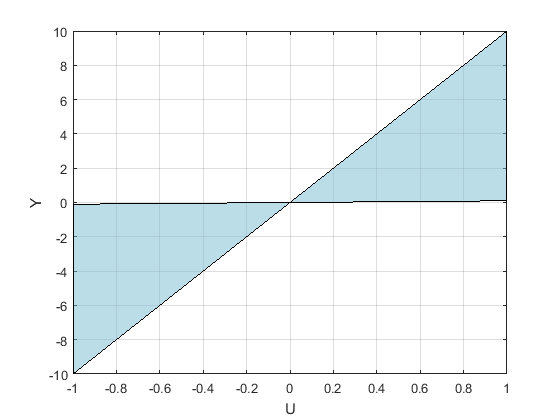The Q matrix for this sector is given by:

```a = 0.1; b = 10; Q = [1 -(a+b)/2 ; -(a+b)/2 a*b];```

A trajectory $y\left(t\right)=G\phantom{\rule{0.1em}{0ex}}u\left(t\right)$ lies within the sector S when for all T > 0,

`$0.1{\int }_{0}^{T}u{\left(t\right)}^{2}<\phantom{\rule{0.2777777777777778em}{0ex}}\phantom{\rule{0.2777777777777778em}{0ex}}{\int }_{0}^{T}u\left(t\right)y\left(t\right)dt<\phantom{\rule{0.2777777777777778em}{0ex}}\phantom{\rule{0.2777777777777778em}{0ex}}10{\int }_{0}^{T}u{\left(t\right)}^{2}dt.$`

In the frequency domain, this same condition can be expressed as:

`${\left(\begin{array}{c}G\left(j\omega \right)\\ 1\end{array}\right)}^{H}Q\left(\begin{array}{c}G\left(j\omega \right)\\ 1\end{array}\right)<0.$`

To check whether `G` satisfies or violates this condition at any frequency, plot the sector index for `H = [G;1]`.

```G = tf([1 2],[1 1]); sectorplot([G;1],Q)```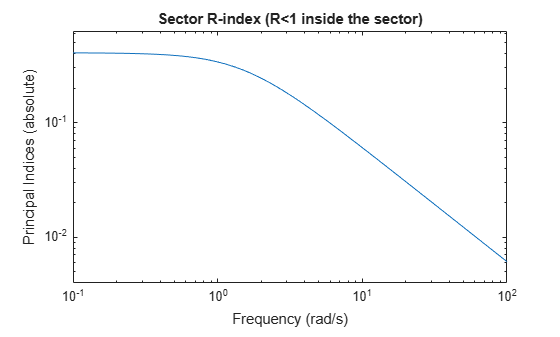The plot shows that the sector index is less than 1 at all frequencies. Therefore, the trajectories of G(s) fit within in the specified sector Q at all frequencies.

Examine the sector plot of a 2-output, 2-input system for a particular sector. Load the system, `H1`, and define the sector, `Q`.

```load("sectorExampleSystem.mat","H1") Q = [-5.12 2.16 -2.04 2.17 2.16 -1.22 -0.28 -1.11 -2.04 -0.28 -3.35 0.00 2.17 -1.11 0.00 0.18];```

Create the plot.

`sectorplot(H1,Q)`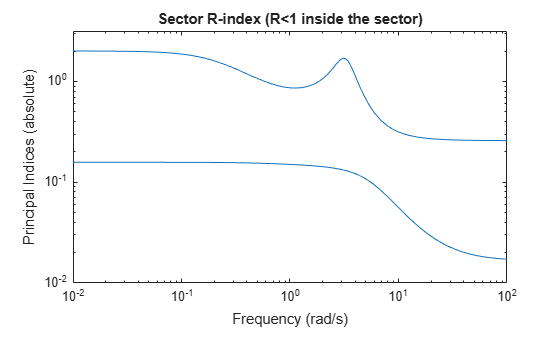Because `H` is 2-by-2, there are two lines on the sector plot. The largest value of the sector index exceeds 1 below about 0.5 rad/s and in a narrow band around 3 rad/s. Therefore, `H` does not satisfy the sector bound represented by `Q`.

Plot the relative sector indices of a model with complex coefficients and a model with real coefficients on the same plot.

```A = [-3.50,-1.25-0.25i;2,0]; B = [1;0]; C = [-0.75-0.5i,0.625-0.125i]; D = 0.5; Hc = [ss(A,B,C,D);1]; % create system with complex coefficients load("sectorExampleSystem.mat","Hr") % load system with real coefficients Q = [1 0.1;0.1 -1]; sectorplot(Hc,Hr,Q) legend('Complex-coefficient model','Real-coefficient model','Location','southwest')```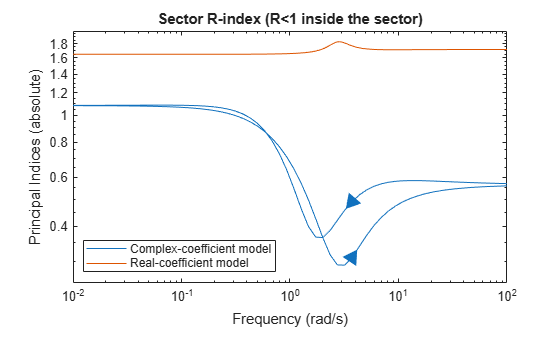In log frequency scale, the plot shows two branches for models with complex coefficients, one for positive frequencies, with a right-pointing arrow, and one for negative frequencies, with a left-pointing arrow. In both branches, the arrows indicate the direction of increasing frequencies. The plots for models with real coefficients always contain a single branch with no arrows.

Set the plotting frequency scale to linear.

```opt = sectorplotoptions; opt.FreqScale = 'Linear';```

Plot the indices.

```sectorplot(Hc,Hr,Q,opt) legend('Complex-coefficient model','Real-coefficient model','Location','southwest')```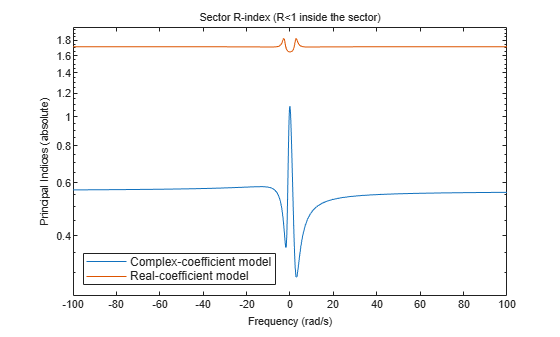In linear frequency scale, the plots show a single branch with a symmetric frequency range centered at a frequency value of zero. The plot also shows the negative-frequency response of a model with real coefficients when you plot the response along with a model with complex coefficients.

## Input Arguments

collapse all

Model to analyze against sector bounds, specified as a dynamic system model such as a `tf`, `ss`, or `genss` model. `H` can be continuous or discrete. If `H` is a generalized model with tunable or uncertain blocks, `sectorplot` analyzes the current, nominal value of `H`.

To analyze whether all I/O trajectories (u(t),y(t) of a linear system G lie in a particular sector, use `H = [G;I]`, where ```I = eyes(nu)```, and `nu` is the number of inputs of `G`.

If `H` is a model array, then `sectorplot` plots the sector index of all models in the array on the same plot. When you use output arguments to get sector-index data, `H` must be a single model.

Sector geometry, specified as:

• A matrix, for constant sector geometry. `Q` is a symmetric square matrix that is `ny` on a side, where `ny` is the number of outputs of `H`.

• An LTI model, for frequency-dependent sector geometry. `Q` satisfies Q(s)’ = Q(–s). In other words, Q(s) evaluates to a Hermitian matrix at each frequency.

The matrix `Q` must be indefinite to describe a well-defined conic sector. An indefinite matrix has both positive and negative eigenvalues.

Frequencies at which to compute and plot indices, specified as the cell array `{wmin,wmax}` or as a vector of frequency values.

• If `w` is a cell array of the form `{wmin,wmax}`, then the function computes the index at frequencies ranging between `wmin` and `wmax`.

• If `w` is a vector of frequencies, then the function computes the index at each specified frequency. For example, use `logspace` to generate a row vector with logarithmically-spaced frequency values.

For models with complex coefficients, if you specify a frequency range of [wmin,wmax] for your plot, then in:

• Log frequency scale, the plot frequency limits are set to [wmin,wmax] and the plot shows two branches, one for positive frequencies [wmin,wmax] and one for negative frequencies [–wmax,–wmin].

• Linear frequency scale, the plot frequency limits are set to [–wmax,wmax] and the plot shows a single branch with a symmetric frequency range centered at a frequency value of zero.

Specify frequencies in units of rad/`TimeUnit`, where `TimeUnit` is the `TimeUnit` property of the model.

Line style, marker, and color, specified as a string or vector of one, two, or three characters. The characters can appear in any order. You do not need to specify all three characteristics (line style, marker, and color). For example, if you omit the line style and specify the marker, then the plot shows only the marker and no line. For more information about configuring this argument, see the `LineSpec` input argument of the `plot` function.

Example: `'r--'` specifies a red dashed line

Example: `'*b'` specifies blue asterisk markers

Example: `'y'` specifies a yellow line

Sector index plot options set, specified as a `SectorPlotOptions` object. You can use this option set to customize the plot appearance. Use `sectorplotoptions` to create the option set. Settings you specify in `plotoptions` overrides the preference settings in the MATLAB session in which you run `sectorplot`. Therefore, `plotoptions` is useful when you want to write a script to generate multiple plots that look the same regardless of the local preferences.

For the list of available options, see `sectorplotoptions`.

## Output Arguments

collapse all

Sector indices as a function of frequency, returned as a matrix. `index` contains the sector indices computed at the frequencies `w` if you supplied them, or `wout` if you did not. `index` has as many columns as there are values in `w` or `wout`, and as many rows as `H` has inputs. Thus the value `index(:,k)` gives the sector indices in descending order at the frequency `w(k)`.

For example, suppose that `G` is a 3-input, 3-output system, `Q` is a suitable sector matrix, and `w` is a 1-by-30 vector of frequencies, then the following syntax returns a 3-by-30 matrix `index`.

```H = [G;eyes(3)] index = sectorplot(H,Q,w);```

The entry `index(:,k)` contains the three sector indices for `H`, in descending order, at the frequency `w(k)`.

`wout` also contains negative frequency values for models with complex coefficients.